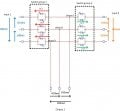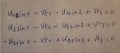# Need your advices on how can I estimate the voltage at the terminals the thyristor switch

#### rachidm

Joined Dec 23, 2018
7I am working on a static transfer switch which aim is to allow supplying a load with 2 different sources in order to have a redundancy when feeding the load. The head to tail assembly allow the current to flow in 2 directions (for each alternative of the input current)

The working condition is the following:

When both input are connected to the supply grid and if we want to supply the load at the output with Input 2 supply, the thyristors of Switch group 1 are openned (off state) and thyristors of switch group 2 will be closed (on state). So that at the output line voltages U12in2, U23in2 and U31in2 are applied.

I am trying to find out an equation that helps to calculate the value of the off state voltage across the thyristor switch Vt1, Vt2 and Vt3 in the following condition:

-Input 1 and input 2 connected to 2 different power supply

-Thyristors of switch group 2 in on state (closed) => triggering signal on so Vt1' = Vt2' = Vt3' =0

-Thyristors of switch group 1 openned (off state) => triggering signal off

I have 3 equations but I am not sure about my results:#### crutschow

Joined Mar 14, 2008
31,493
Do the two sources have the same phase?

#### rachidm

Joined Dec 23, 2018
7
Do the two sources have the same phase?
Thank you for your reply Crutschow. There is a phase shift of 180°. I have forgotten to mention it.

#### MrAl

Joined Jun 17, 2014
9,761
View attachment 230203

I am working on a static transfer switch which aim is to allow supplying a load with 2 different sources in order to have a redundancy when feeding the load. The head to tail assembly allow the current to flow in 2 directions (for each alternative of the input current)

The working condition is the following:

When both input are connected to the supply grid and if we want to supply the load at the output with Input 2 supply, the thyristors of Switch group 1 are openned (off state) and thyristors of switch group 2 will be closed (on state). So that at the output line voltages U12in2, U23in2 and U31in2 are applied.

I am trying to find out an equation that helps to calculate the value of the off state voltage across the thyristor switch Vt1, Vt2 and Vt3 in the following condition:

-Input 1 and input 2 connected to 2 different power supply

-Thyristors of switch group 2 in on state (closed) => triggering signal on so Vt1' = Vt2' = Vt3' =0

-Thyristors of switch group 1 openned (off state) => triggering signal off

I have 3 equations but I am not sure about my results:

View attachment 230205

Hi,

This is interesting because i designed a 100 amp static transfer switch back in the mid 1980's. I used a high speed ROM to handle the input state to gate drive logic so it was a fairly compact design even for back then.

But your question can always be investigated using some simple expressions for sine waves like:
Vdiff=A*sin(wt+ph1)-B*sin(wt+ph2)

where the two sine expressions are simply the expressions for each side of any two terminals in the circuit. You can go three phase by simply setting the phase shifts as needed.

If any two sine waves are in phase though then there wont be any difference. If they are 180 degrees out of phase then there will be maximum difference of Vpeak1+Vpeak2 because one side will be maximum positive and the other maximum negative and so the difference is Vpeak1-(-Vpeak2) which is simply the sum of the two peak measurements. So for example if you have 170v peak for both waves then the max difference will be 340v when the two are at their positive and negative peaks.

If the two sines are subject to a varying phase difference then the max peak is still the same as 180 degrees except that condition may occur less often as the two change relative to each other.

In the design i did i had to incorporate amplitude measurements, current measurements, and phase measurements into the algorithm. There were other required specs too but i dont remember all of that now it was many years ago. This was for a commercial application that was to be used for many different inverter projects that had to have backup AC power.

I invite you to look at the simple expressions above for two sine waves as you vary the phase difference it is quite a simple concept.